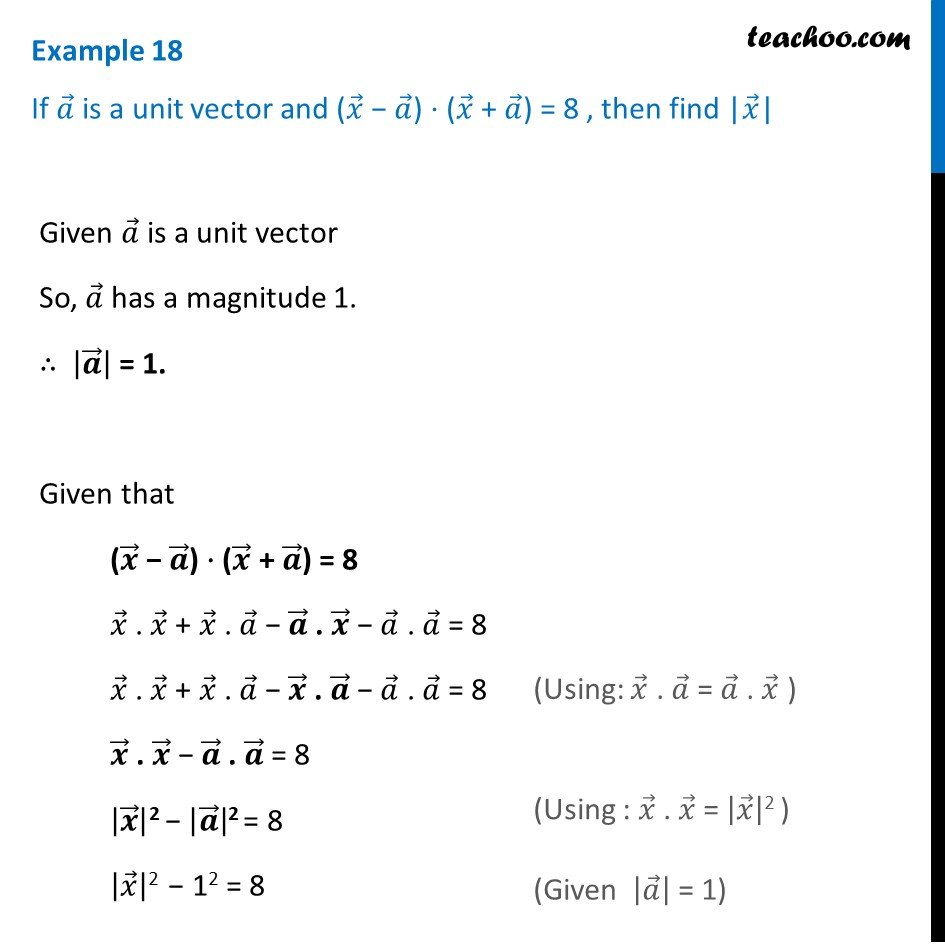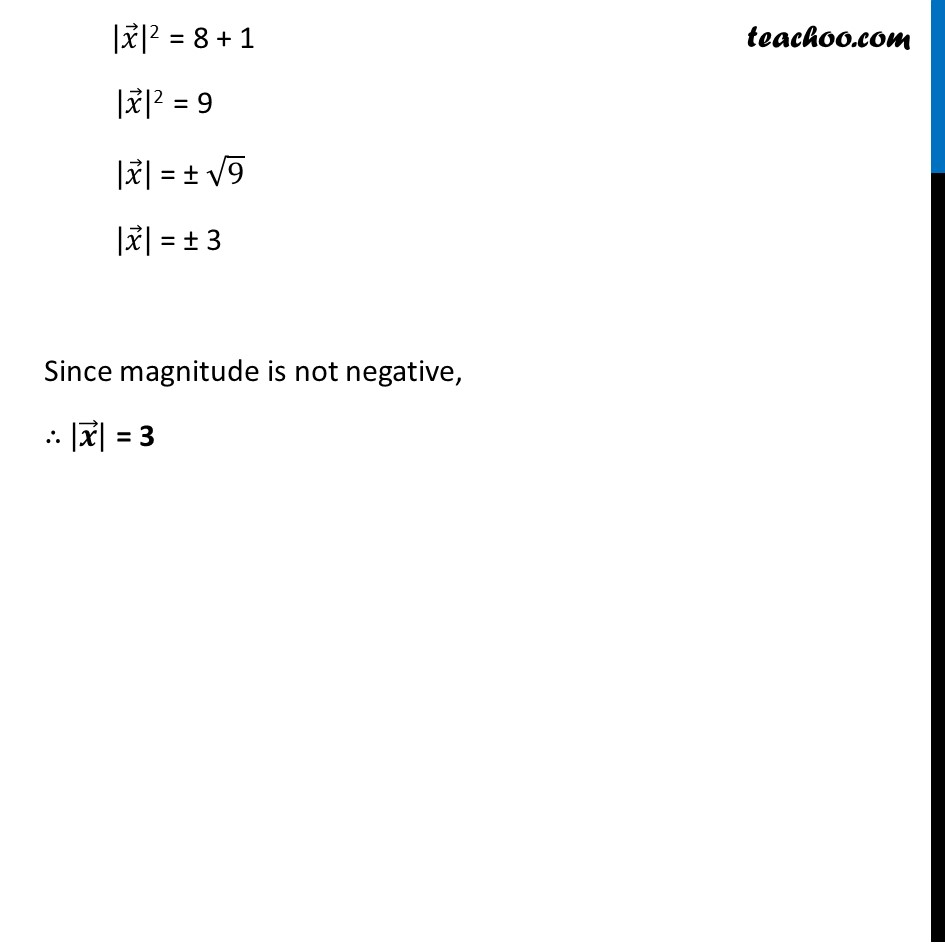Examples

Chapter 10 Class 12 Vector Algebra
Serial order wiseGet live Maths 1-on-1 Classs - Class 6 to 12

### Transcript

Example 18 If 𝑎 ⃗ is a unit vector and (𝑥 ⃗ − 𝑎 ⃗) ⋅ (𝑥 ⃗ + 𝑎 ⃗) = 8 , then find |𝑥 ⃗| Given 𝑎 ⃗ is a unit vector So, 𝑎 ⃗ has a magnitude 1. ∴ |𝒂 ⃗ | = 1. Given that (𝒙 ⃗ − 𝒂 ⃗) ⋅ (𝒙 ⃗ + 𝒂 ⃗) = 8 𝑥 ⃗ . 𝑥 ⃗ + 𝑥 ⃗ . 𝑎 ⃗ − 𝒂 ⃗ . 𝒙 ⃗ − 𝑎 ⃗ . 𝑎 ⃗ = 8 𝑥 ⃗ . 𝑥 ⃗ + 𝑥 ⃗ . 𝑎 ⃗ − 𝒙 ⃗ . 𝒂 ⃗ − 𝑎 ⃗ . 𝑎 ⃗ = 8 𝒙 ⃗ . 𝒙 ⃗ − 𝒂 ⃗ . 𝒂 ⃗ = 8 |𝒙 ⃗ |2 − |𝒂 ⃗ |2 = 8 |𝑥 ⃗ |2 − 12 = 8 (Using: 𝑥 ⃗ . 𝑎 ⃗ = 𝑎 ⃗ . 𝑥 ⃗ ) |𝑥 ⃗ |2 = 8 + 1 |𝑥 ⃗ |2 = 9 |𝑥 ⃗ | = ± √9 |𝑥 ⃗ | = ± 3 Since magnitude is not negative, ∴ |𝒙 ⃗ | = 3